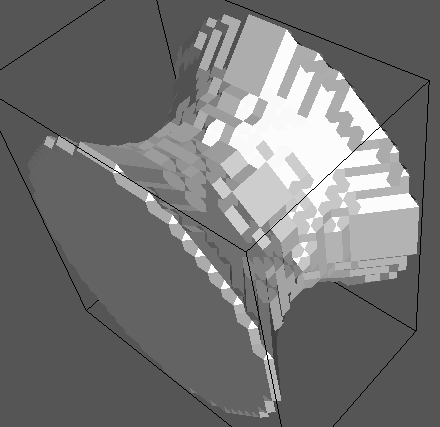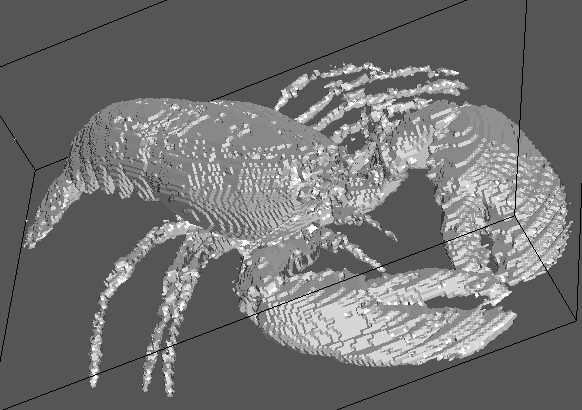DGtal  1.3.beta
topology/volToOFF.cpp

Marching-cube like surface extracted using the combinatorial manifold structure of digital surfaces.

Application to export surface in OFF format

On the lobser.vol volume, volToOFF.cpp builds an OFF surface of 155068 vertices, 154910 faces, 310136 edges in 3879ms+1646ms.

* # Commands
* $./examples/topology/volToOff ../examples/samples/cat10.vol 1 255 0 *$ ./examples/topology/volToOff ../examples/samples/lobster.vol 50 255 0
*Marching-cube surface of cat10.vol file.Marching-cube surface of lobster.vol file.
#include <iostream>
#include "DGtal/base/Common.h"
#include "DGtal/helpers/StdDefs.h"
#include "DGtal/topology/helpers/Surfaces.h"
#include "DGtal/topology/DigitalSurface.h"
#include "DGtal/topology/SetOfSurfels.h"
#include "DGtal/images/ImageSelector.h"
#include "DGtal/images/imagesSetsUtils/SetFromImage.h"
using namespace std;
using namespace DGtal;
using namespace Z3i;
void usage( int, char** argv )
{
std::cerr << "Usage: " << argv[ 0 ] << " <fileName.vol> <minT> <maxT> <int=0|ext=1>" << std::endl;
std::cerr << "\t - displays the boundary of the shape stored in vol file <fileName.vol> as an OFF geomview surface file. It is a kind of marching-cube surface, defined by duality with respect to the digital surface." << std::endl;
std::cerr << "\t - voxel v belongs to the shape iff its value I(v) follows minT <= I(v) <= maxT." << std::endl;
std::cerr << "\t - 0: interior adjacency, 1: exterior adjacency." << std::endl;
}
int main( int argc, char** argv )
{
if ( argc < 5 )
{
usage( argc, argv );
return 1;
}
std::string inputFilename = argv[ 1 ];
unsigned int minThreshold = atoi( argv[ 2 ] );
unsigned int maxThreshold = atoi( argv[ 3 ] );
bool intAdjacency = atoi( argv[ 4 ] ) == 0;
trace.beginBlock( "Reading vol file into an image." );
DigitalSet set3d (image.domain());
minThreshold, maxThreshold);
// Construct the Khalimsky space from the image domain
bool space_ok = K.init( image.domain().lowerBound(),
image.domain().upperBound(), true );
if (!space_ok)
{
trace.error() << "Error in the Khamisky space construction."<<std::endl;
return 2;
}
trace.beginBlock( "Extracting boundary by scanning the space. " );
typedef SetOfSurfels< KSpace, SurfelSet > MySetOfSurfels;
Surfaces<KSpace>::sMakeBoundary( theSetOfSurfels.surfelSet(),
K, set3d,
image.domain().lowerBound(),
image.domain().upperBound() );
MyDigitalSurface digSurf( theSetOfSurfels );
trace.info() << "Digital surface has " << digSurf.size() << " surfels."
<< std::endl;
trace.beginBlock( "Making OFF surface <marching-cube.off>. " );
ofstream out( "marching-cube.off" );
if ( out.good() )
digSurf.exportSurfaceAs3DOFF( out );
out.close();
return 0;
}
DGtal::Surfaces
Aim: A utility class for constructing surfaces (i.e. set of (n-1)-cells).
Definition: Surfaces.h:78
DGtal::SetFromImage
Aim: Define utilities to convert a digital set into an image.
Definition: SetFromImage.h:63
DGtal::Trace::endBlock
double endBlock()
DGtal::KhalimskySpaceND::SurfelSet
std::set< SCell > SurfelSet
Preferred type for defining a set of surfels (always signed cells).
Definition: KhalimskySpaceND.h:450
DGtal::DigitalSurface
Aim: Represents a set of n-1-cells in a nD space, together with adjacency relation between these cell...
Definition: DigitalSurface.h:139
DGtal::Trace::error
std::ostream & error()
DGtal::KhalimskySpaceND::init
bool init(const Point &lower, const Point &upper, bool isClosed)
Specifies the upper and lower bounds for the maximal cells in this space.
DGtal::trace
Trace trace
Definition: Common.h:154
K
KSpace K
Definition: testCubicalComplex.cpp:62
DGtal::Trace::beginBlock
void beginBlock(const std::string &keyword="")
DGtal::KhalimskySpaceND::lowerBound
const Point & lowerBound() const
Return the lower bound for digital points in this space.
DGtal::Trace::info
std::ostream & info()
DGtal::Z2i::DigitalSet
DigitalSetSelector< Domain, BIG_DS+HIGH_BEL_DS >::Type DigitalSet
Definition: StdDefs.h:100
Image
ImageContainerBySTLVector< Domain, Value > Image
Definition: testSimpleRandomAccessRangeFromPoint.cpp:45
DGtal
DGtal is the top-level namespace which contains all DGtal functions and types.
main
int main(int argc, char **argv)
Definition: testArithmeticDSS-benchmark.cpp:147
DGtal::Image
Aim: implements association bewteen points lying in a digital domain and values.
Definition: Image.h:69
usage
void usage(int, char **argv)
Definition: approximation.cpp:64
Aim: implements methods to read a "Vol" file format.